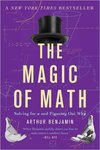## All HMC Faculty Books#### Title

The Magic of Math: Solving for x and Figuring Out Why

#### Description

The Magic of Math is the math book you wish you had in school. Using a delightful assortment of examples-from ice-cream scoops and poker hands to measuring mountains and making magic squares-this book revels in key mathematical fields including arithmetic, algebra, geometry, and calculus, plus Fibonacci numbers, infinity, and, of course, mathematical magic tricks. Known throughout the world as the "mathemagician," Arthur Benjamin mixes mathematics and magic to make the subject fun, attractive, and easy to understand for math fan and math-phobic alike.

0465096212

2015

Basic Books

New York

#### Keywords

Mathematics, Algebra, Geometry, Calculus, Fibonacci

#### Disciplines

Algebra | Geometry and Topology | MathematicsCOinS